Normal view MARC view ISBD view

# A SAS/IML companion for linear models / Jamis J. Perrett.

Material type:TextSeries: Statistics and computing: Publisher: New York : Springer, c2010Description: xiv, 228 p. : ill. ; 24 cm.ISBN: 9781441955562 (pbk. : acidfree paper); 1441955569 (pbk. : acidfree paper); 1441955577 (ebk.); 9781441955579 (ebk.).DDC classification: 519.535 Online resources: WorldCat details | E-book Fulltext
Contents:
Table of contents SAS/IML: A Brief Introduction.- IML Language Structure.- IML Programming Features.- Matrix Manipulations in SAS/IML.- Mathematical and Statistical Basics.- Linear Algebra.- The Multivariate Normal Distribution.- The General Linear Model.- Linear Mixed Models.- Statistical Computation Methods.
Summary: Linear Models Courses are often presented as either theoretical or applied. This book bridges the gap between the derivation of formulas and analyses that hide these formulas. It includes complete examples of SAS code as well as code for high-level procedures.
Tags from this library: No tags from this library for this title.Average rating: 0.0 (0 votes)
Item type Current location Collection Call number Copy number Status Date due Barcode Item holdsE-Book
E-book
Non-fiction 519.535 PES 2010 (Browse shelf) Not for loanText
Reserve Section
Non-fiction 519.535 PES 2010 (Browse shelf) C-1 Not For Loan 26642
Total holds: 0
##### Browsing EWU Library Shelves , Shelving location: Reserve Section Close shelf browser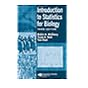No cover image available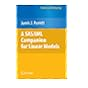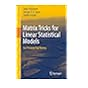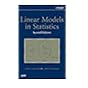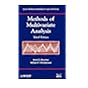519.535 MCI 2007 Introduction to statistics for biology / 519.535 MUL 2019 Multivariate data analysis / 519.535 NEI 2006 An introduction to copulas / 519.535 PES 2010 A SAS/IML companion for linear models / 519.535 PUM 2011 Matrix tricks for linear statistical models : 519.535 REL 2008 Linear models in statistics / 519.535 REM 2012 Methods of multivariate analysis /

Includes bibliographical references and index.

Table of contents SAS/IML: A Brief Introduction.- IML Language Structure.- IML Programming Features.- Matrix Manipulations in SAS/IML.- Mathematical and Statistical Basics.- Linear Algebra.- The Multivariate Normal Distribution.- The General Linear Model.- Linear Mixed Models.- Statistical Computation Methods.

Linear Models Courses are often presented as either theoretical or applied. This book bridges the gap between the derivation of formulas and analyses that hide these formulas. It includes complete examples of SAS code as well as code for high-level procedures.

Applied Statistics Mathematics and Physical Sciences

There are no comments for this item.